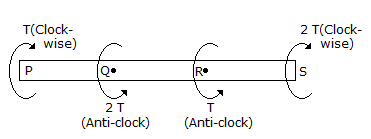# Civil Engineering - UPSC Civil Service Exam Questions

36.

Probability of a lO-year flood to occur at least once in the next 4 years is

 A. 25% B. 35% C. 50% D. 65%

Explanation:

No answer description available for this question. Let us discuss.

37.

Weigh-batching proceeds on

 A. the assumption of the declared weight in each bag of cement B. weighing the contents of each bag C. accurately estimating the weight of each material to be used in each batch D. the assumption of correct dry weight of each size range of each material and the weight of water.

Explanation:

No answer description available for this question. Let us discuss.

38.

A cantilever beam of span l carries a uniformly varying load of zero intensity at the free end and w per metre length at the fixed end. What does the integration of the ordinate of the load diagram between the limits of free and fixed ends of the beam give ?

 A. Bending moment at the fixed end B. Shear force at the fixed end C. Bending moment at the free end D. Shear force at the free end

Explanation:

No answer description available for this question. Let us discuss.

39.

A shaft PQRS is subjected to torques at P, Q, R, S as shown in the given figure.
The maximum torque for the shaft section occurs betweenA. P and Q B. P and R C. Q and R D. R and S

Explanation:

No answer description available for this question. Let us discuss.

40.

When the recirculation ratio in a high rate trickling filter is unity, then what is the value of the recirculation factor ?

 A. 1 B. > 1 C. < 1 D. Zero